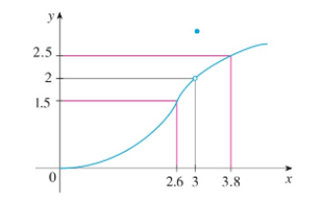Chapter 2.4, Problem 2E### Single Variable Calculus: Early Tr...

8th Edition
James Stewart
ISBN: 9781305270343

#### Solutions

Chapter
Section### Single Variable Calculus: Early Tr...

8th Edition
James Stewart
ISBN: 9781305270343
Textbook Problem

# Use the given graph of f to find a number δ such thatif 0<|x –3|< δ then |f(x)– 2 |< 0.5To determine

To find: The value of a number δ by using the graph of f.

Explanation

Given:

If 0<|x3|<δ, then |f(x)2|<0.5.

Definition used:

If for every number ε>0 there is a number δ>0 such that if 0<|xa|<δ, then |f(x)L|<ε.

Absolute value inequality: If |x|<a,a>0 then a<x<a.

Calculation:

Consider |f(x)2|<0.5.

By absolute value definition, 0.5<f(x)2<0.5.

Simplify the inequality by adding 2 on each side,

20.5<2+f(x)2<2+0

### Still sussing out bartleby?

Check out a sample textbook solution.

See a sample solution

#### The Solution to Your Study Problems

Bartleby provides explanations to thousands of textbook problems written by our experts, many with advanced degrees!

Get Started